# Simpleton 2002 - The Workshop### 8. - 12. July 2002

Centre International de Rencontres Mathématiques
Luminy, Marseilles
FranceI should like to thank all speakers and participants at Simpleton 2002 - The Workshop. I hope you have enjoyed the meeting as much as I have.

You can find the definitive programme below, as well as the updated list of abstracts. I shall add links to the slides of the talks in the abstract section when and if I get them from the speakers. Eventually, all talks except for Ziegler's (and the corresponding slides, if any) will be available here on the CIRM website. Finally, here is the conference photo.

### Programme

TimeMondayTuesdayWednesday ThursdayFriday
7h00 - 9h00Breakfast
9h15 - 10h15Casanovas HartWagner Ben-Yaacov Chatzidakis
10h15 - 10h45Coffee Break
10h45 - 11h45Onshuus KimTomasic Pourmahdian Shami
11h55 - 12h25Iwanow de PiroLippel EvansKrupinski
12h30Lunch
14h00 - 14h30  Ealy
14h35 - 15h30Newelski
16h15 - 17h15Kikyo Newelski   BerensteinCoffee Break
17h15 - 17h45Coffee Break Coffee Break
17h45 - 18h45MacPherson ZieglerPillay
18h55 - 19h25Problem SessionTsuboi Ben-Yaacov
19h30Dinner

### Tutorials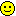Enrique Casanovas : Simplicity simplified We present the basics of simplicity theory, up to the independence theorem. The proofs will use several simplifications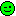found recently by different people, which may also be of interest for experts. Bradd Hart : Hyperimaginaries and canonical bases Shelah's eq-construction gives a canonical first-order way to deal with classes modulo definable equivalence relations, which is all we need in the stable case. In the simple context things may no longer be so simple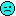: The general theory requires us to consider classes modulo type-definable equivalence relations, so-called hyperimaginaries - although there is no first-order example where hyperimaginaries cannot be eliminated in favour of ordinary imaginaries. I shall develop the model theory of hyperimaginaries, and present their most important occurrence, namely as canonical base for a Lascar strong type. Frank Wagner : Groups in simple theories Slides I shall present the basic properties of simple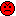groups (normal subgroups, chain conditions, generic types, Schlichting's Theorem and variants). Itaï Ben-Yaacov : Simplicity in compact abstract theories Slides When working with simple theories, one may get the impression that the distinction between formulas and partial types is not so important (in contrast with the stable case). In other words, the ability to take negation is not so important, but compactness is. We turn this impression into a precise statement by working in the framework of compact abstract theories (cats): negation is taken out, but compactness is preserved for positive formulas. For the sake of simplicity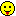we add the (very weak) assumption of thickness, namely that indiscernibility is type-definable (with positive formulas). I will describe the framework of thick cats, and how first order simplicity theory generalises to it fully, although some of the proofs need to be modified. Examples of thick simple (or stable) cats include: simple (or stable) first order/Robinson theories, Hilbert spaces, etc. Zoé Chatzidakis : Simplicity in fields I will recall some of the classical results on fields with a simple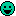theory, and then present examples of such fields, maybe with additional structure.

### Abstracts

Itaï Ben-Yaacov: Non first-order lovely pairs Slides
Abstract: This is a continuation of Anand Pillay's talk about lovely pairs, and describes an offshoot of common work with him and Evgueni Vassiliev.
We observe that for every (complete) simple theory T there is a (unique) theory TP whose saturated models are precisely the lovely pairs of models of T: it just may happen that TP is not first order, but rather a compact abstract theory (cat). In fact, we do not need to assume that T is first order, and the construction goes through for every thick simple cat. Properties such as simplicity, supersimplicity, stability, and others are preserved.
I will sketch the proof of this result, and use it as an example for several notions that arise naturally when considering simple cats.

Alexander Berenstein: Dividing in the algebra of compact operators over a Hilbert space
Abstract: Simplicity and Stability inside a Hilbert space H can be studied from many points of view. One approach is looking at H as a large strongly homogeneous structure and apply the tools developed by Buechler and Lessmann. We review some of the properties of independence and dividing from this perspective inside a Hilbert space. We also define the algebra of finite rank operators on H and of compact operators on H. We study simplicity and stability inside these structures and the relationship with independence in H.

Tristam de Piro: The geometry of minimal types in simple theories
Abstract: I will present a geometric proof that linearity implies one-basedness for rank 1 Lascar strong types, and summarise closely related results.

Clifton Ealy: þ-forking in simple theories
Abstract: I sketch the proof that þ-forking is the same as forking in a simple theory with elimination of hyperimaginaries.

David Evans: Ample dividing
Abstract:We construct a stable universal domain in which forking is trivial, but which has a reduct which is n-ample for all n, with respect to a reasonably well-behaved notion of independence. In particular, the reduct is not CM-trivial. It is not clear whether the structure (and therefore the reduct) is actually stable in the full first-order sense.

Alexander Iwanow: Strongly determined types and simple theories
Abstract: The notion of a strongly determined type generalizes the notion of a definable type. In a stable theory every type extends to a strongly determined one. The question when this is true in a simple theory is central in the talk. In particular I am going to discuss the case of simple theories obtained from stable ones by adding a relation in some generic way.

Kikyo, Hirotaka: Generic automorphisms of unstable theories
Abstract: I will talk on the conjecture stating that if T is unstable and model complete, then the theory T + `ø is an automorphism' does not have a model companion.
The conjecture is true if T has the strict order property, or if T has the amalgamation property for automorphisms.
Allthough the first example for the conjecture was the theory of the random graph, I unfortunately still don't have a general argument valid for simple theories.

Byunghan Kim: Non-finite axiomatizability of some w-categorical rank-1 theories
Abstract: We study the non-finite axiomatizability of w-categorical rank-1 structures. We reach the desired conclusion for the case of a trivial geometry, and for a generic predicate. Extensions beyond the rank-1 level are expected. We conjecture the result for any w-categorical 1-based theory.
(Joint work with de Piro and Young.)

Krzysztof Krupinski: Profinite structures interpretable in separably closed fields
Abstract: Profinite structures are inverse limits of finite permutational structures. We consider the question which profinite structures are interpretable in separably closed fields. We introduce the notion of profinite structures weakly interpretable in a first-order theory. Using some model theory of separably closed fields (and showing some new results) we show that profinite structures interpretable in separably closed fields are the same as profinite structures weakly interpretable in ACFp. We then find a strong connection between the last notion and the the main problem of Galois theory. We also construct examples with particular model-theortic properties (smallness, m-normality, non-triviality, or M-rank 1).

David Lippel: What w-categorical theories are finitely axiomatizable ?
Abstract: I shall survey results on finite axiomatizability of w-categorical theories.

Dugald Macpherson: Asymptotics of definable sets in finite structures
Abstract: A theorem of Chatzidakis, van den Dries and Macintyre gives good asymptotic information on the sizes of definable sets in finite fields, and yields a notion of measure (and dimension) for definable sets in pseudofinite fields. I will describe joint work with Charles Steinhorn, in which we consider arbitrary classes of finite structures which satisfy the conclusion of the CDM theorem. This leads to the notion of `measurable' supersimple theory, which will be discussed.

Ludomir Newelski: Local modularity in simple theories
Abstract: I will recall the definition of local modularity in stable theories and will discuss this concept in the simple case. I will show how to generalize the weakly minimal case to some supersimple theories.

Ludomir Newelski: The diameter of a Lascar strong type
Abstract: Let R be the reflexive, symmetric and type-definable relation which holds of (a0, a1) if a0, a1,... start an infinite indiscernible sequence, and let d(a, b)=n be the minimal length of an R-chain a = a0 R a1 R ... R an = b, and infinite if no such chain exists. Given a Lascar strong type X, put diam(X)=sup { d(a, b) : a, b in X }. We show that diam(X) is finite iff X is type-definable; moreover, any type-definable union of Lascar strong types of infinite diameter contains at least continuum many Lascar strong types. The proofs use the new topological tool of an open analysis of a compact space with respect to a given covering.

Alf Onshuus: Properties and consequences of þ-independence Slides
Abstract: We develop a new notion of independence, þ-independence, and show that in a large class of theories (including all simple ones) it has many of the relevant geometric properties. In stable, supersimple and o-minimal theories it agrees with the usual notion of independence, thus giving a unified approach to stability (simplicity) and o-minimality. Finally, we discuss some connections between þ-independence and the stable forking conjecture.

Anand Pillay: Lovely pairs of models of a simple theory
Abstract: We study a simple analogue of Poizat's belles paires, called lovely pairs. We give criteria for a saturated model of the theory TP of lovely pairs (of models of a simple theory T) to be also a lovely pair. The criteria provide an analogue of nonfcp for simple theories. In this case, TP is also simple and we study forking and canonical bases in TP. We also prove that T is low iff the class of lovely pairs is Robinson.
(Joint work with Itaï Ben-Yaacov and Evgueni Vassiliev.)

Massoud Pourmahdian: A simple homogeneous model without the stable forking property
Abstract: We use Fraïssé-Hrushovski amalgamation to construct a simple homogeneous model without the stable forking property.

Ziv Shami: Unidimensional simple theories
Abstract: We will present some recent coordinatisation theorems and new foreignness notions for simple theories, and some of their applications to unidimensional simple theories.

Ivan Tomasic: The group configuration in simple theories and geometric simplicity theory
Abstract: We shall give a survey of the recent work with Ben-Yaacov and Wagner on the group configuration in simple theories and its applications reminiscent of geometric stability theory.

Tsuboi, Akito: Simple theories without PAPA.
Abstract: I will give some examples of simple theories without PAPA. I also state some problems concerning such theories.

Martin Ziegler: A new approach to Lascar strong types
Abstract: The title says it all. Or maybe it doesn't. Oh well - this abstract was written by the organizer anyway.

### List of open problems

• (Bradd Hart) Let T be simple. Is there always a reduct T0 of T such that
• T0 is stable, and
• for all models M0, M1, M2 of T, if M1 and M2 depend over M0, then this forking is witnessed by a (stable) formula in the language of T0 ?
False for pseudofinite fields.

(Itaï Ben-Yaacov) Is there a map from the type-space of T to the type-space of a stable theory preserving independence both ways ?
This is true for Psf, TP, TA.

• (Anand Pillay) Is there an unstable theory (simple, supersimple) with a unique theory of proper pairs ?

• (Ziv Shami) Let U be an Ø-invariant (large) set. Call a type p controlled over U if there is a set A of parameters such that all realizations of p are in DCL(U, A). Put Û = { a : tp(a) is controlled over U }, the controlled hull of U.
• Determine the possibilities for Û in a simple theory.
• If I(U) = { a : tp(a) is U-internal }, does Û contain I(U) in a simple theory ?

• (David Evans) Let M be w-categorical of SU-rank 1 with weak elimination of imaginaries. Suppose A1, A2, A3,... is a chain of algebraically closed subsets of M with dim(Ai) = i. Let li be the minimal line size when localizing M at Ai. Note that li is non-decreasing.
Can li go to infinity ?
Observations
• If there is some q such that |acl(a1, a2,..., am)| < qm, then li is eventually constant.
• If li is eventually constant at least 3, we get a group configuration.

### List of participants

Florence Azé (Lyon-1)
Itaï Ben-Yaacov (Paris-7)
Alexander Berenstein (Notre Dame)
Thomas Blossier (Paris-7)
Elisabeth Bouscaren (CNRS, Paris-7)
Enrique Casanovas Ruiz-Fornells (Barcelona)
Zoé Chatzidakis (CNRS, Paris-7)
Tristam De Piro (MIT)
Françoise Delon (CNRS, Paris-7)
Clifton Ealy (UC Berkeley)
David Evans (East Anglia)
Martin Hils (Paris-7)
Alexander Iwanow (Woclaw)
Kikyo, Hirotaka (Tokai)
Byunghan Kim (MIT)
Martin Körwien (Paris-7)
Piotr Kowalski (Wroclaw)
Krzysztof Krupinski (Wroclaw)
David Lippel (McMaster)
Angus Macintyre (Edinburgh)
Dugald Macpherson (Leeds)
Yerulan Mustafin (Lyon-1)
Ludomir Newelski (Wroclaw)
Herwig Nubling (East Anglia)
Alf Onshuus (UC Berkeley)
Assaf Peretz (UC Berkeley)
Anand Pillay (UI Urbana-Champaign)
Françoise Point (FNRS, Mons-Hainaut)
Juan Francisco Pons i Llopis (Barcelona)
Massoud Pourmahdian (East Anglia)
Serge Randriambobolona (ENS Lyon)
Cédric Rivière (Mons-Hainaut)
Olivier Roche (Lyon-1)
Ziv Shami (McMaster)
Katrin Tent (Würzburg)
Ivan Tomasic (Leeds)
Tsuboi, Akito (Tsukuba)
Alex Usvyatsov (Jerusalem)
Frank Wagner (Lyon-1)
Roman Wencel (Wroclaw)
Jessica Young (MIT)
Martin Ziegler (Freiburg)Frank O. Wagner

Institut Girard Desargues
et
Institut universitaire de France# Logicum Lugdunensis

La page du thème logique